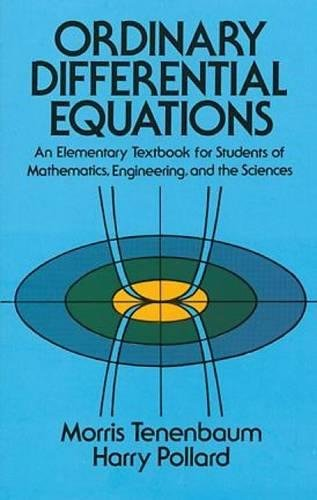Please Enter ISBN, Title or Author’s Nameand more...

# Ordinary Differential Equations Dover Books on Mathematics | Revised ed. editionAuthors: Morris Tenenbaum,Harry Pollard
ISBN:9780486649405
ISBN-13: 9780486649405
List Price: \$26.95 (up to 84% savings)
Prices shown are the lowest from
the top textbook retailers.

## View all Prices by Retailer## Details about Ordinary Differential Equations Dover Books on Mathematics:

This unusually well-written, skillfully organized introductory text provides an exhaustive survey of ordinary differential equations — equations which express the relationship between variables and their derivatives. In a disarmingly simple, step-by-step style that never sacrifices mathematical rigor, the authors — Morris Tenenbaum of Cornell University, and Harry Pollard of Purdue University — introduce and explain complex, critically-important concepts to undergraduate students of mathematics, engineering and the sciences.
The book begins with a section that examines the origin of differential equations, defines basic terms and outlines the general solution of a differential equation-the solution that actually contains every solution of such an equation. Subsequent sections deal with such subjects as: integrating factors; dilution and accretion problems; the algebra of complex numbers; the linearization of first order systems; Laplace Transforms; Newton's Interpolation Formulas; and Picard's Method of Successive Approximations.
The book contains two exceptional chapters: one on series methods of solving differential equations, the second on numerical methods of solving differential equations. The first includes a discussion of the Legendre Differential Equation, Legendre Functions, Legendre Polynomials, the Bessel Differential Equation, and the Laguerre Differential Equation. Throughout the book, every term is clearly defined and every theorem lucidly and thoroughly analyzed, and there is an admirable balance between the theory of differential equations and their application. An abundance of solved problems and practice exercises enhances the value of Ordinary Differential Equations as a classroom text for undergraduate students and teaching professionals. The book concludes with an in-depth examination of existence and uniqueness theorems about a variety of differential equations, as well as an introduction to the theory of determinants and theorems about Wronskians.

Need a Differential Equations tutor? View profile below:
Roohollah E.
(4 reviews)
Education: Silver Spring MD
Major: Professional Math Tutor

I have a PhD in Math from Yale. I am currently a faculty member at the University of Maryland, College Park. As a student I won a Silver Medal in the International Math Olympiad (1996 IMO). As a coach, I have extensive experience training students for math competitions, both at high school and college level. I have taught math at different levels. Currently I only work with motivated students who are interested in learning math beyond the usual school curriculum. I can help you prepare for ... Read more

I have a PhD in Math from Yale. I am currently a faculty member at the University of Maryland, College Park. As a student I won a Silver Medal in the International Math Olympiad (1996 IMO). As a coach, I have extensive experience training students for math competitions, both at high school and college level. I have taught math at different levels. Currently I only work with motivated students who are interested in learning math beyond the usual school curriculum. I can help you prepare for ... Read more

Need Differential Equations course notes? Start your search below: